Introduction to computational neurosciencePurpose of the study track
This study track is intended for those with either a background in neurobiology or informatics looking to gain a basic understanding of computational neuroscience.

Computational neuroscience is a branch of neuroscience which employs mathematical models, theoretical analysis and abstractions of the brain to understand the principles that govern the development, structure, physiology, and cognitive abilities of the nervous system.

Most who enter the field of computational neuroscience have a prior background in either mathematics, physics, computer science, or (neuro)biology. Since computational neuroscience requires a bit of knowledge from all these fields, with some basic knowledge of neurons and a familiarity with certain types of equations and mathematical concepts, we recommend two different "starting tracks" depending on the student's background before you begin the lectures listed below:

##### Lectures in this study track
1This lecture covers an Introduction to neuron anatomy and signaling, and different types of models, including the Hodgkin-Huxley model.

2This lecture describes non-spiking simple neuron models used in artificial neural networks and machine learning.

3

Introduction to simple spiking neuron models.

4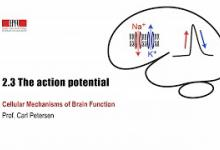The ionic basis of the action potential, including the Hodgkin Huxley model.

5Forms of plasticity on many levels - short-term, long-term, metaplasticity, structural plasticity. With examples related to modelling of…

6Introduction to modelling of chemical computation in the brain

7Conference presentation on computationally demanding studies of synaptic plasticity on the molecular level

8Introduction to stability analysis of neural models

9Introduction to stability analysis of neural models

10Oscillations and bursting

11Oscillations and bursting

12Weakly coupled oscillators

13Continuation of coupled oscillators

14Firing rate models.

15Pattern generation in visual system hallucinations.

16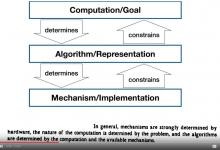Introduction to the role of models in theoretical neuroscience

17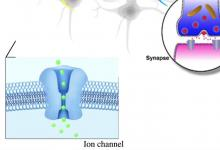Different types of models, model complexity, and how to choose an appropriate model.

18Balanced E-I networks, stability and gain modulation

19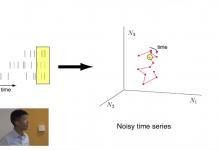Methods for dimensionality reduction of data, with focus on factor analysis.

20Methods for dimensionality reduction of data, with focus on factor analysis.

21Spiking neuron networks and linear response models.

22Bayesian neuron models and parameter estimation.

23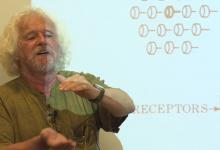Constraints can help us understand how the brain works.

24Approaching neural systems from an evolutionary perspective

##### Courses in this study track
1

Introductory lectures on different aspects of biochemical models

2

Introductory lectures on different aspects of Biophysical models.

3

The CAJAL computational neuroscience courses teaches the central ideas, methods, and practice of modern computational neuroscience through a…

5

Introductory lectures on different aspects of biochemical models.

6

Introductory lectures on different aspects of statistical models.